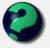# Math Is Fun Forum

Discussion about math, puzzles, games and fun.   Useful symbols: ÷ × ½ √ ∞ ≠ ≤ ≥ ≈ ⇒ ± ∈ Δ θ ∴ ∑ ∫  π  -¹ ² ³ °

You are not logged in.

## #1 2006-04-09 11:43:06

vgopalak
Member
Registered: 2006-04-05
Posts: 3

### A simple puzzle

A car travels from city A to city B. After two hours they had a problem and hence reduced the speed to 30 mph. As a result they were late by an hour. If they faced the problem 15 miles further down, they would have been 40 minutes late. Determine the distance between the two cities.

Offline

## #2 2006-04-09 16:53:53

ganeshRegistered: 2005-06-28
Posts: 34,580

### Re: A simple puzzle

225 milesIt is no good to try to stop knowledge from going forward. Ignorance is never better than knowledge - Enrico Fermi.

Nothing is better than reading and gaining more and more knowledge - Stephen William Hawking.

Offline

## #3 2006-04-14 05:01:20

John E. Franklin
MemberRegistered: 2005-08-29
Posts: 3,588

### Re: A simple puzzle

90 MPH is very fast!!  I wonder what highway that was?

igloo myrtilles fourmis

Offline

## #4 2008-06-30 18:23:19

bpraveen02
Member
Registered: 2008-06-30
Posts: 0

### Re: A simple puzzle

I would like to know the solutions for this problem.
Can u please provide me the solultion?

Offline

## #5 2008-07-01 00:14:28

mathsyperson
ModeratorRegistered: 2005-06-22
Posts: 4,900

### Re: A simple puzzle

The important things here are the distance from the problem to the destination, and the speed that the car was going at before having the problem. (The distance from the start to the destination will eventually be important, but that can easily be found once we know the initial speed).

Say the initial speed is "v" mph , and the distance travelled after having the problem is "d" miles.

The time taken to reach the city after having the problem is given by

.
However, if there wasn't a problem then it would have been
.

The car is one hour late, and so the difference between these times must be one hour:

The second statement is that if the problem had been 15 miles further up, then the delay would have been 40 minutes (ie. 2/3 of an hour). If this was the case, then the distance from the problem to the city would have been 15 less, and so we have:

These are two simultaneous equations and two variables, so both variables can be found (although it is a bit messy).

Rearranging equation I:

Now put this value of v into equation II:

And tidy up:

We now know the distance from the city when the problem was encountered.

Putting this into the expression for v gives:

Checking the values of d = 45; v = 90 with the original equations shows that they do indeed work.

The last thing to consider is that the car was travelling for 2 hours pre-problem. We now know that this was at 90mph, so the distance covered in that time was 180 miles.
Add this to the 45 miles travelled after the problem, and you get the final answer of 225 miles.

Why did the vector cross the road?
It wanted to be normal.

Offline